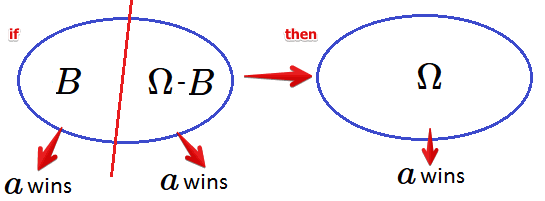# ML Wiki

## Separability

The monotonicity is a Voting Theory principle that characterizes voting methods for choosing the winner.The separability principle is satisfied if

• when we split a region $\Omega$ into subregions $B$ and $\Omega - B$ and run the election in both
• if the same candidate $a$ wins in both sub regions $B$ and $\Omega - B$
• then $a$ should win if the election were run for the whole region $\Omega$

Also, when considering the whole rankings:

• suppose for $B$ the ranking is $a_1 > ... > a_n$ and for $\Omega - B$ the ranking is $a_1 > ... a_n$
• then for $\Omega$ the ranking should also be $a_1 > ... > a_n$

It this criterion is not satisfied, then we can split the region into subregions in such a way that we achieve the desired outcome (i.e. it may lead to manipulation)

## Methods

Methods that respect Separability:

Methods that don't respect Separability: